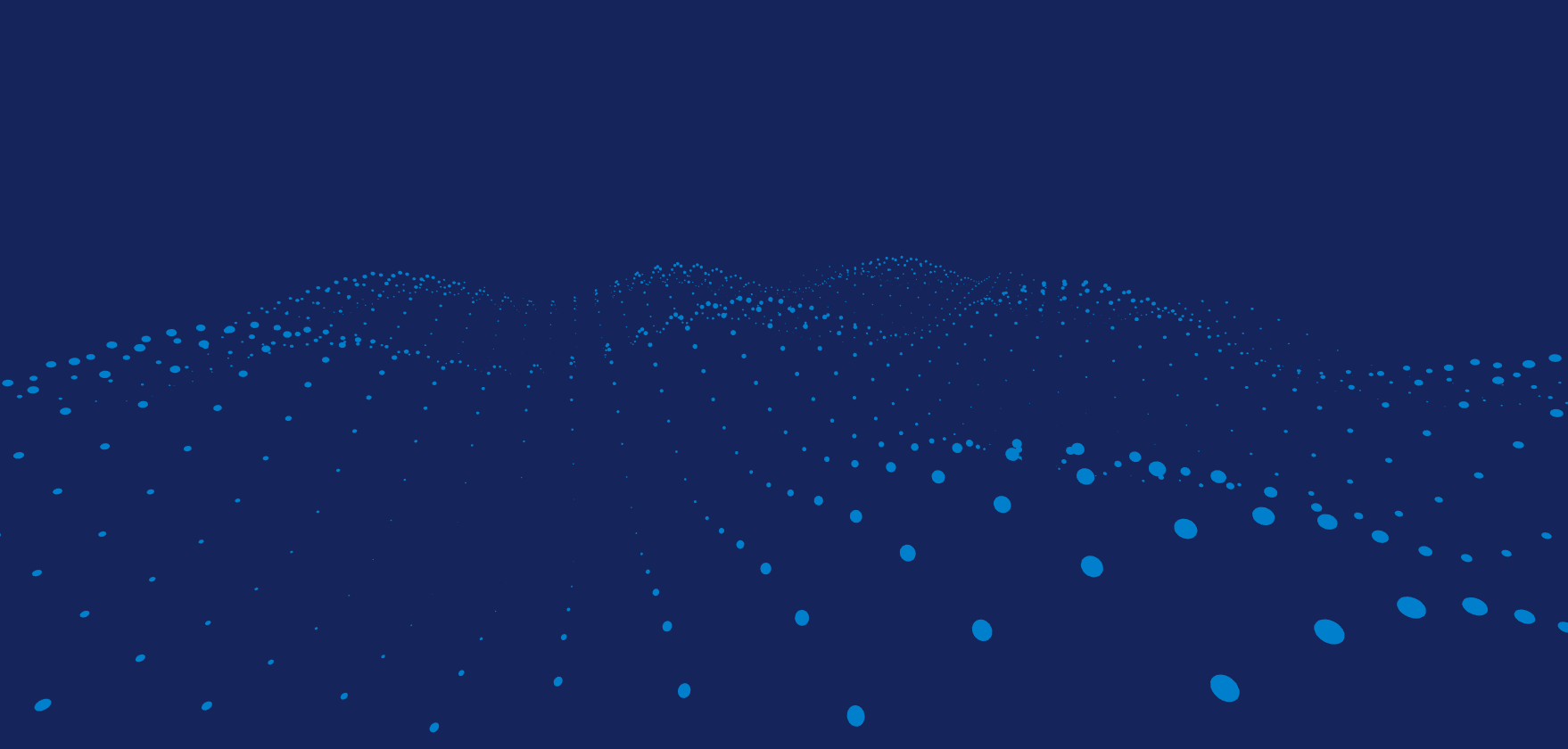# three.js 着色器材质之变量（二）

「查缺补漏」巩固你的Redis知识体系

`attributes should now be defined in THREE.BufferGeometry instead.`《RabbitMQ》如何保证消息不被重复消费

## 1. 制作几何体

```let geometry = new THREE.Geometry();
for(let i=0; i<39; i++) { //39行
for(let j=0; j<39; j++) { //39列
let sphere = new THREE.SphereGeometry(2, 15, 10);
sphere.translate(i * 50 - 950, 0, j * 50 - 950); //这里我们使用Geometry的translate方法将间隔调成50
geometry.merge(sphere);//结合几何体
}
}
let bufferGeometry = new THREE.BufferGeometry().fromGeometry(geometry); //最后将几何体替换成对应的缓冲类型```

## 2. 设置attributes属性

```total = bufferGeometry.attributes.position.count; //几何体点的个数
every = total / 39 /39; //每个球体点的个数
centers = new Float32Array(total * 3);//初始化类型数组，并设置长度
//将中心点保存到centers中
for(let i=0; i<39; i++) {
for(let j=0; j<39; j++) {
for(let k=0; k<every; k++) {
centers[(i*39*every + j*every + k) * 3] = i * 50 - 950;
centers[(i*39*every + j*every + k) * 3 + 1] = 0;
centers[(i*39*every + j*every + k) * 3 + 2] = j * 50 - 950;
}
}
}
bufferGeometry.setAttribute('centers', new THREE.BufferAttribute(centers, 3));//为bufferGeometry设置属性```

## 3. 设置着色器材质

```uniforms = {
time: {
type: 'f',
value: 0
}
}
uniforms: uniforms,
attribute vec3 centers;
uniform float time;
void main() {
float centery = sin(centers.x / 100.0 + time) * 40.0 + sin(centers.z / 100.0 + time) * 40.0;
vec3 center = vec3(centers.x, 0, centers.z);
vec3 target = position - center;
vec3 newPosition = center + target * ((centery + 80.0) / 80.0);
gl_Position = projectionMatrix * modelViewMatrix * vec4( newPosition.x, newPosition.y + centery, newPosition.z, 1.0 );
}
`,
void main() {
gl_FragColor = vec4(0.0,0.5,0.8,1.0);
}
`
});```

three.js 着色器材质之变量（二）

【深度思考】如何优雅告知用户，网站正在升级维护？